# Deque Data Structure

### What is Deque?

• Double Ended Queue which is also called Deque is a type of queue data structure in which  insertion and deletion of elements can be either in front or rear.
• It doesn’t follow First In First Out (FIFO) rule
• We will be using a Circular Array for implementing Deque, if the array is FULL , we again start from the beginning . The first element is considered as the next of the Last element .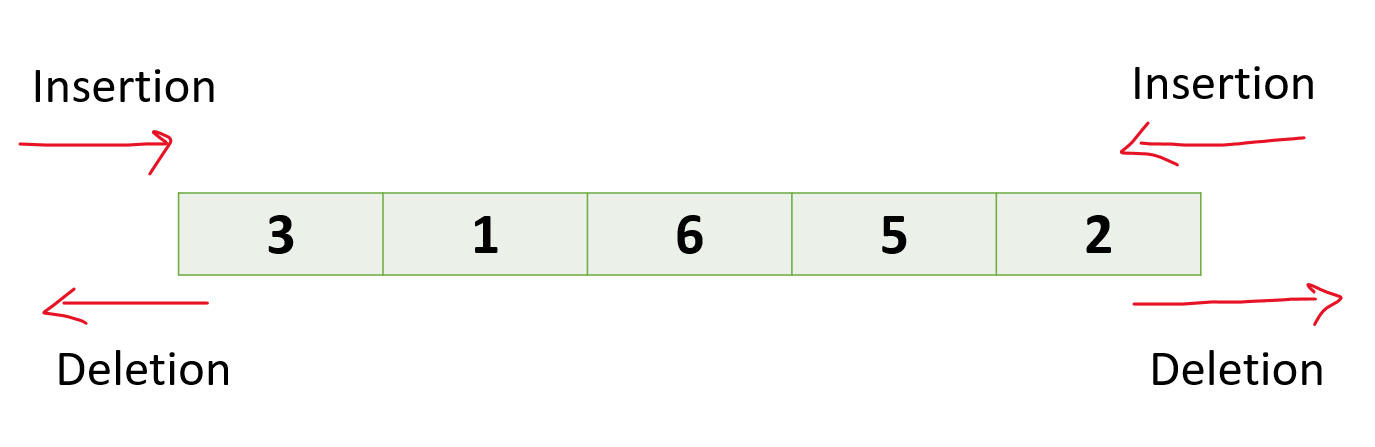### Types of Deque:

There are 2 types of Deque:

1. Input Restricted Queue: Insertion can only be performed at any one end but deletion can be done from both the ends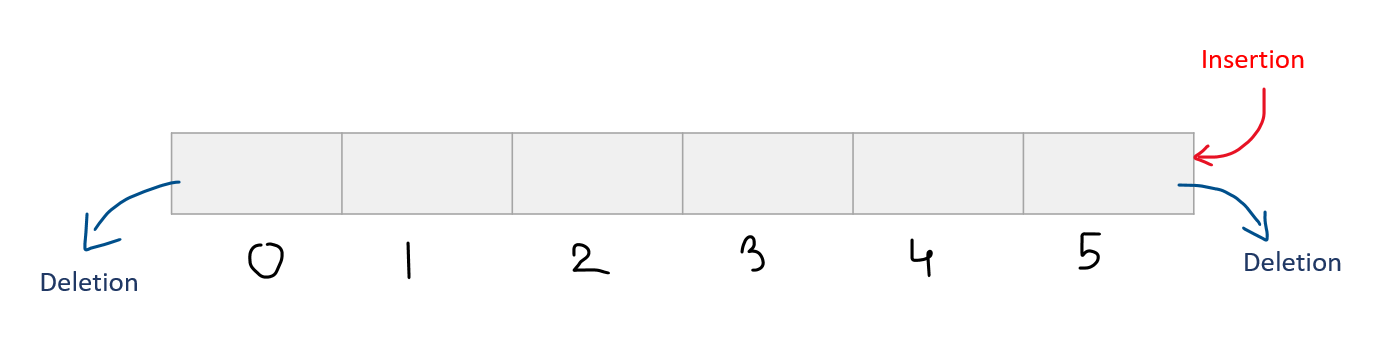2. Output Restricted Queue:  Insertion can only be performed from both ends but deletion can be done from any one end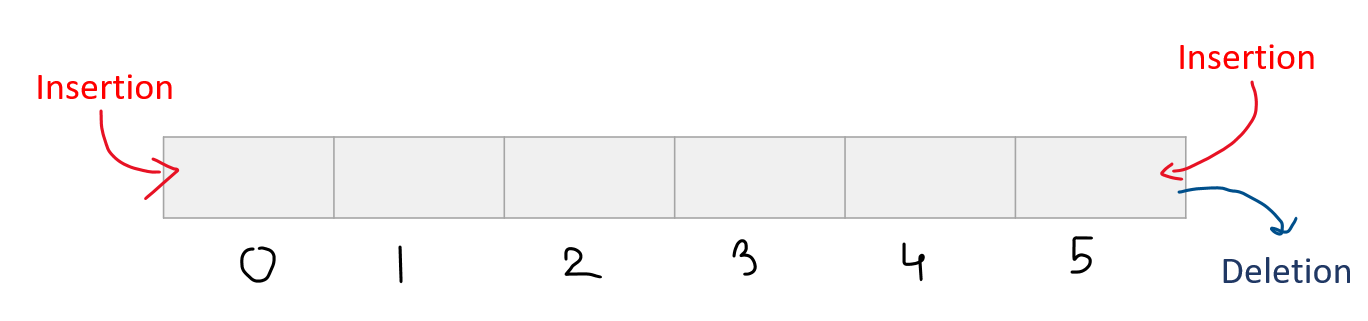### Operations that can be applied on a given deque:

1. Delete an element from the front
2. Delete an element from the rear
3. Insert an element from the front
4. Insert an element from the rear
5. Get the front item from deque
6. Get the rear element from deque
7. Check whether deque is full or not
8. Check whether deque is empty or not

Front – It is used to refer to the first element of the deque. By using it we can fetch the first element of a deque

Rear – It is used to refer to the last element of the deque. By using it we can fetch the last element of a deque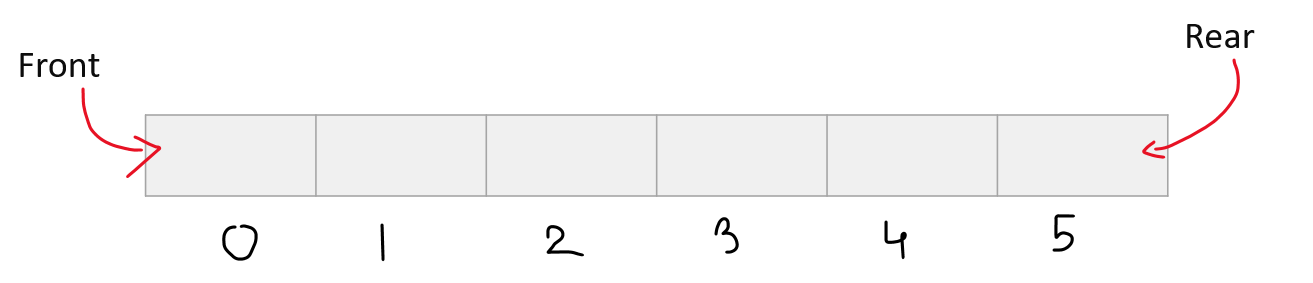Before performing the above-mentioned operations, we will do the following steps :

1. Declare an Array of size N. This array will be used as a deque
2. Set 2 pointers at the first position where Front = -1 and Rear = 0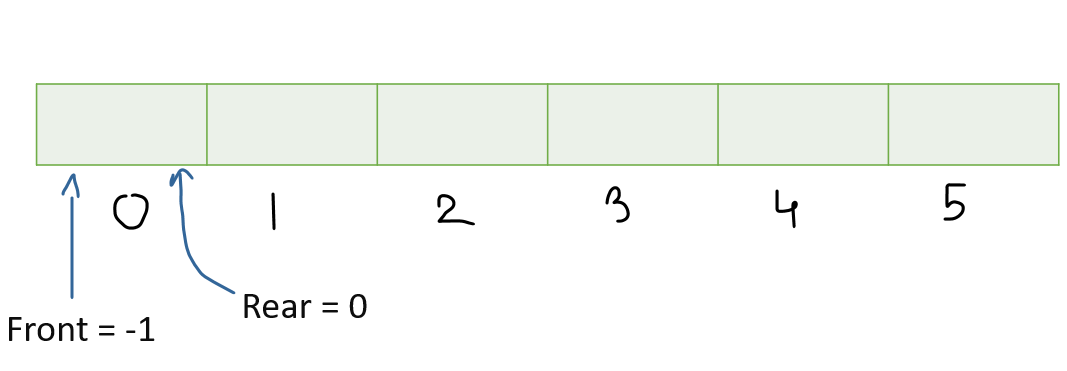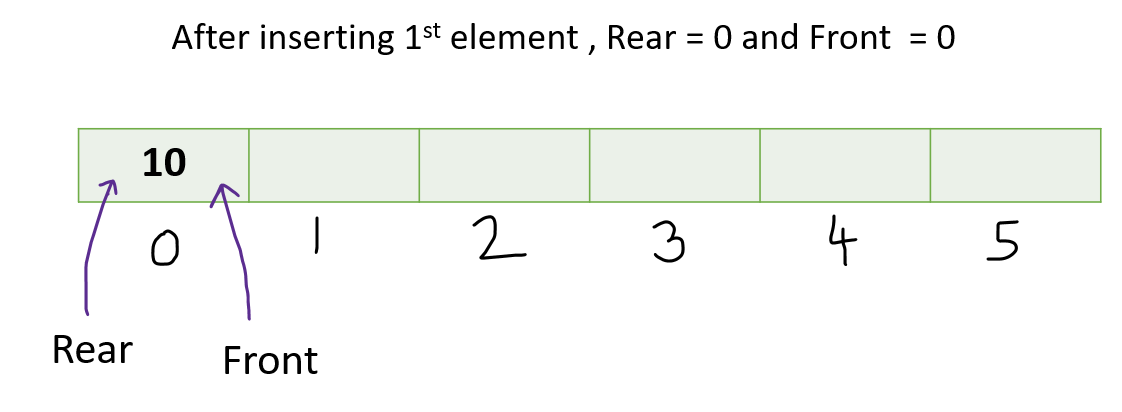## Insert at the Front

Algorithm:

• Check the position of the front
• If front < 1 , reinitialize front = N-1 ( last index )
• Then add new element to Array[front]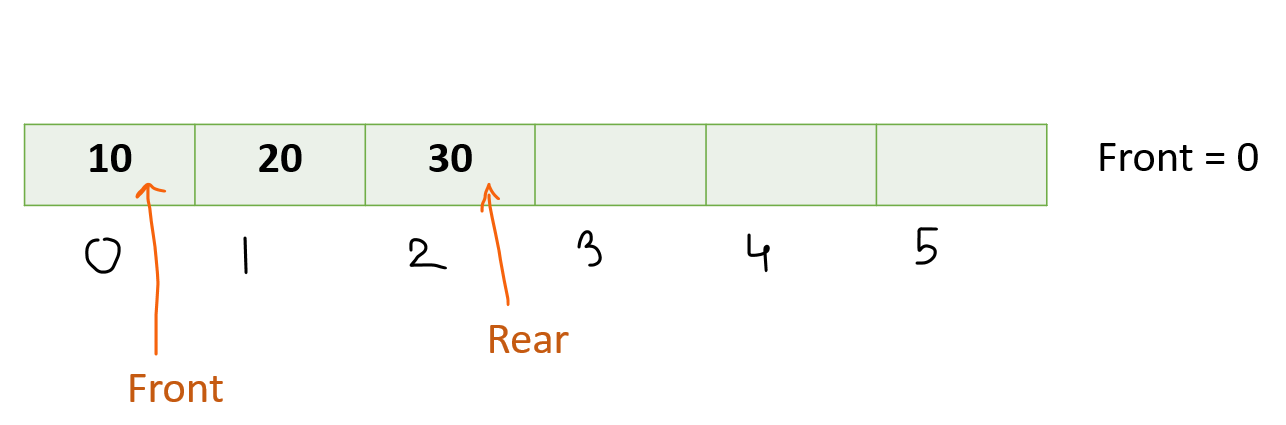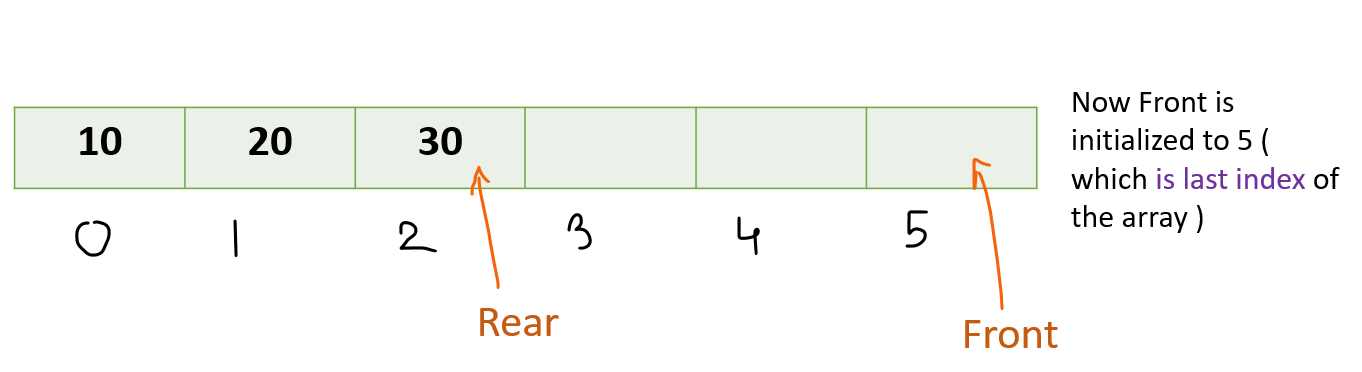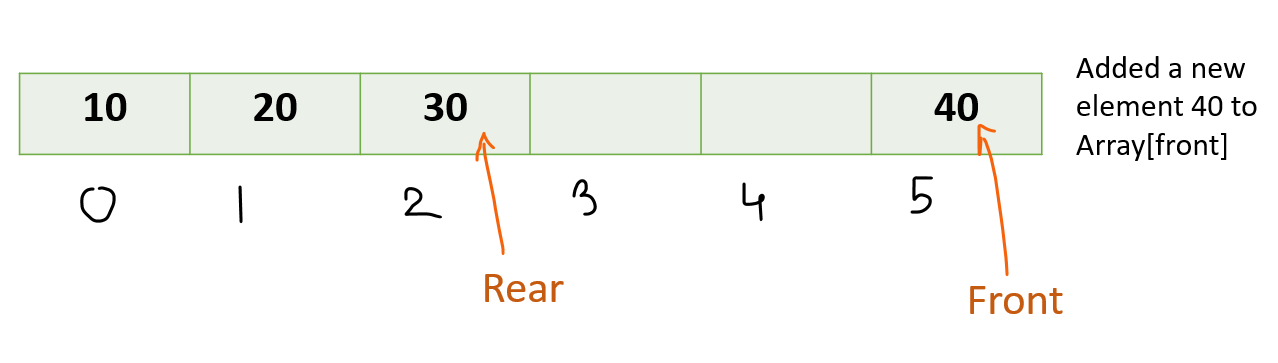## Insert at Rear

Algorithm:

• Check if the array is full or not
• If it’s full , reinitialize rear = 0 else increase rear by 1.
• Then add the element to Array[rear]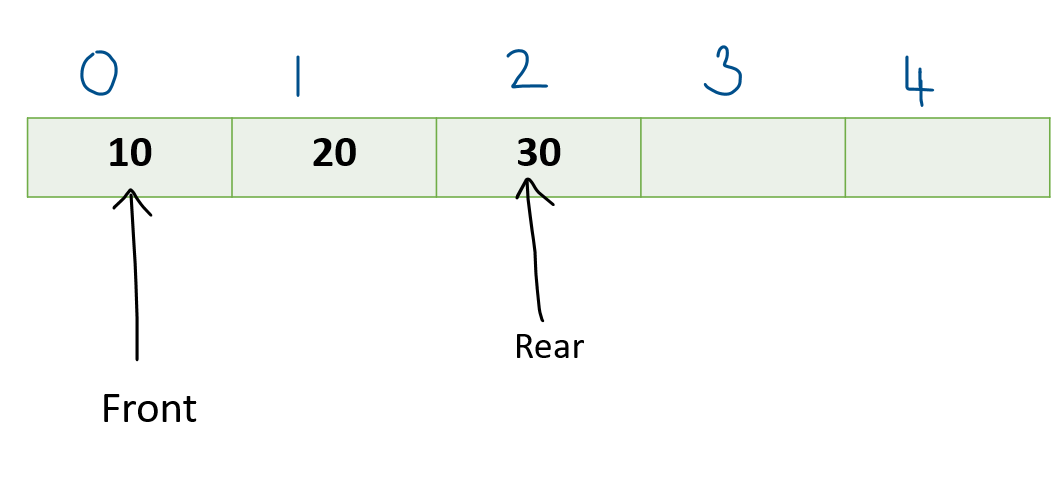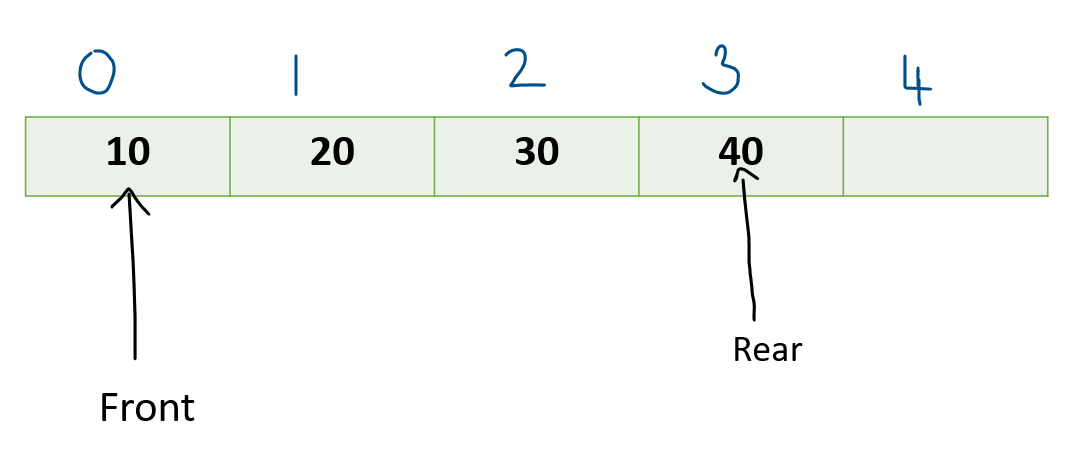## Delete from Front

Algorithm:

• Check if deque is Empty or not Empty.
• If deque is Empty, then deletion operation cannot be performed . In this case , we will simply print UNDERFLOW. In this condition , the front will be -1.
• If deque has only 1 element , then only 1 operation of deletion is possible. In case of 1 element , front == rear.
• We will set front =-1 and rear = -1
• If the front is at the last index ( front == n-1 ) , set front = 0.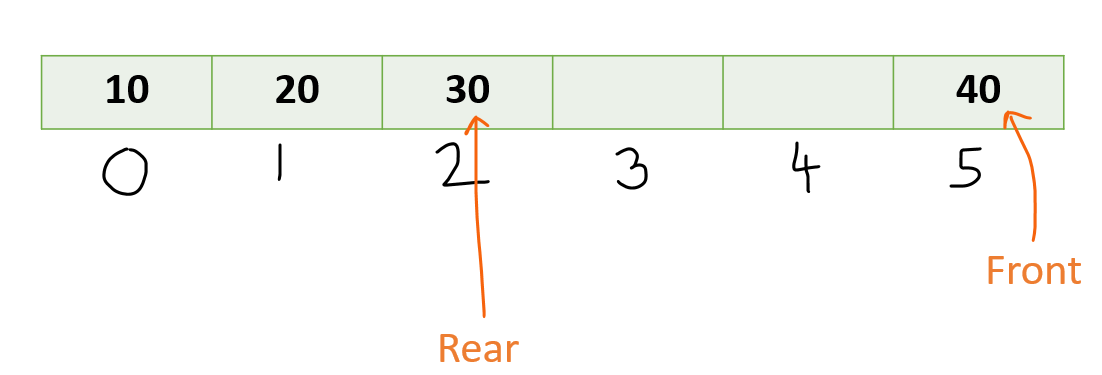• Else set Front = Front + 1.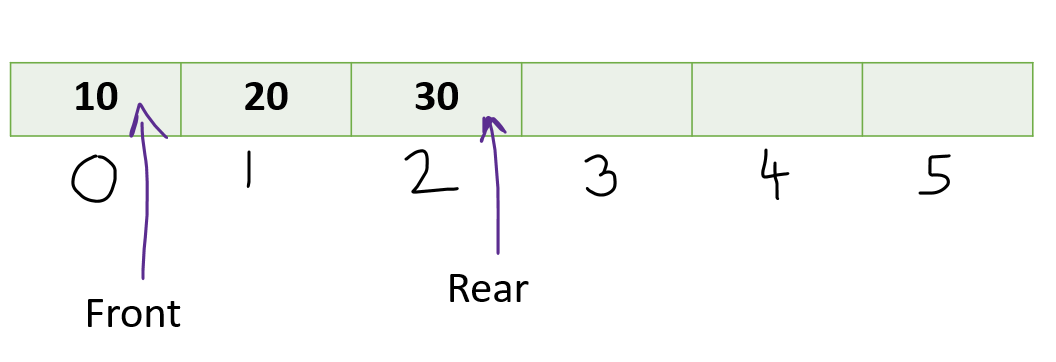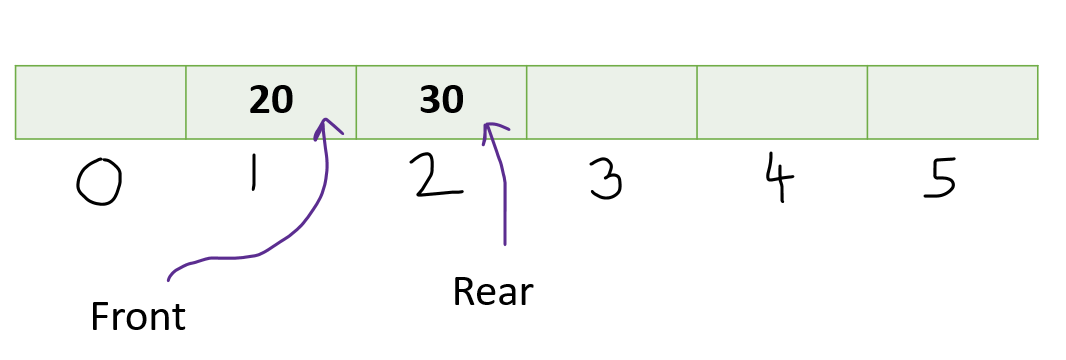## Delete from Rear

Algorithm:

• Check if the deque is empty or not empty. If it’s empty, then we cannot perform a deletion operation. We will directly print UNDERFLOW.
• If the deque has only 1 element i.e front==rear, we will set front = -1 and rear =-1
• If rear is at 0 , then set rear = n-1
• Else rear = rear-1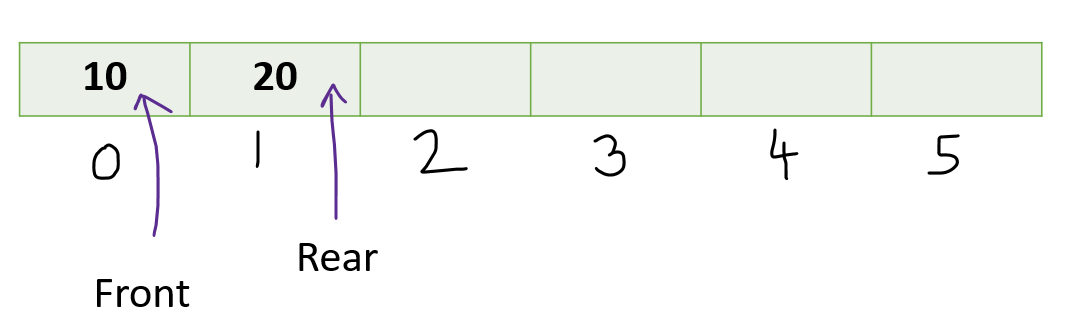• Check if Deque is Empty or Not Empty

If front = -1, then deque is empty else it’s not empty.

• Check if Deque is Full or Not Full

If front == 0 and rear == n-1 , then deque is Full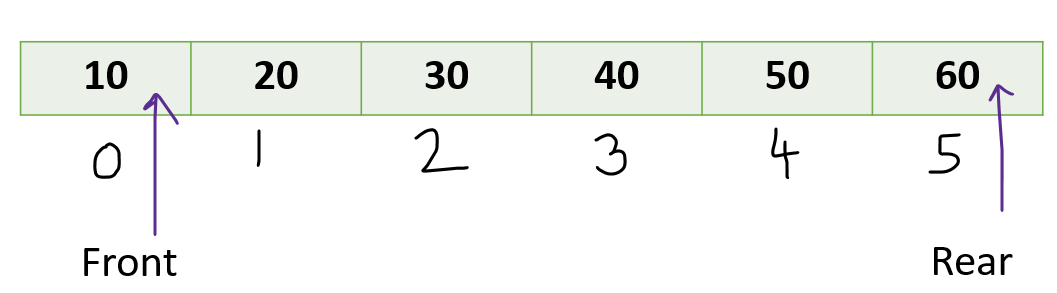->If front == rear + 1 , then also deque is Full.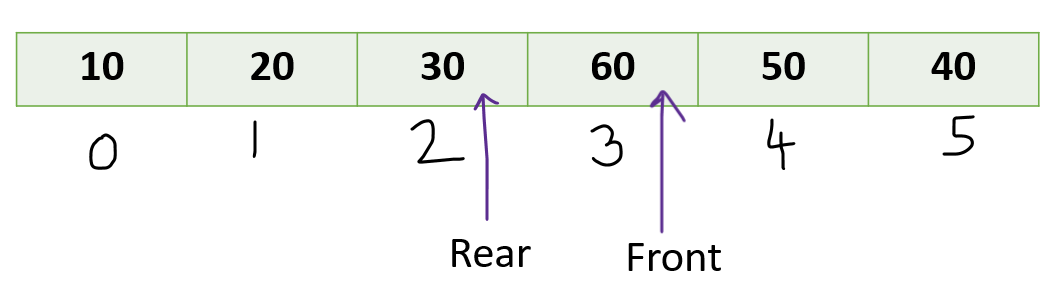->If the deque is Full ,then any new element cannot be inserted . We will directly print OVERFLOW.

Code:

## C++ Code

``````#include <bits/stdc++.h>
using namespace std ;
#define MAX 100

class Deque {
int array[MAX]  ;
int rear, front, size ;

public:
Deque(int size) {
front = -1 ;
rear = 0 ;
this->size = size ;
}

void InsertFront(int element)  ;
void InsertRear(int element)  ;
void DeleteFront()  ;
void DeleteRear()  ;
int GetFront();
int GetRear()  ;
bool isFull() ;
bool isEmpty()  ;
} ;

bool Deque :: isFull() {

if (front == 0 && rear == size - 1) return 1 ;
if (front == rear + 1 ) return 1 ;

return 0 ;
}

bool Deque :: isEmpty() {
if (front == -1) return 1 ;
return 0  ;
}
void Deque::InsertRear(int element) {
if (isFull()) {
cout << "Overflow\n"  ;
return  ;
}

if (rear == size - 1) {
rear = 0 ;
}
else if (front == -1) {
rear = 0 ;
front = 0 ;
}
else {
rear++ ;
}

array[rear] = element  ;
}

void Deque::InsertFront(int element) {
if (isFull()) {
cout << "Overflow\n"  ;
return  ;
}

if (front == 0) {
front = size - 1;
}
else if (front == -1) {
rear = 0 ;
front = 0 ;
}
else {
front-- ;
}

array[front] = element  ;
}

void Deque :: DeleteFront() {

if (isEmpty()) {
cout << "Underflow\n"  ;
return  ;
}

if (front == size - 1) {
front = 0 ;
}
else if (front == rear) {
rear = -1 ;
front = -1;
}
else {
front++ ;
}
}

void Deque::DeleteRear() {
if (isEmpty()) {
cout << "Underflow\n"  ;
return  ;
}

if (rear == 0) {
rear = size - 1;
}
else if (front == rear) {
rear = -1 ;
front = -1;
}
else {
rear-- ;
}
}

int Deque::GetRear() {
if (rear < 0 ) {
cout << "Underflow\n"   ;
return -1 ;
}

if (isEmpty()) {
cout << "Underflow\n"  ;
return -1;
}

return array[rear]  ;
}

int Deque::GetFront() {

if (isEmpty()) {
cout << "Underflow\n"  ;
return -1;
}

return array[front]  ;
}

int main() {
Deque dq(30);

cout << "Inserting element at front end \n";
dq.InsertFront(100);

cout << "Getting front element " << " " << dq.GetFront() << "\n";

dq.DeleteFront();

cout << "After deleting front element new front will be " << dq.GetFront()
<< endl;

cout << "Inserting element at rear end  : 50 \n";
dq.InsertRear(50);

cout << "Inserting element at rear end : 10 \n";
dq.InsertRear(10);

cout << "Rear element " << dq.GetRear() << endl;

dq.DeleteRear();
cout << "After deleting rear element new rear will be " << dq.GetRear() <<
"\n";

return 0 ;
}
``````

Time Complexity : Operations like InsertRear(), InsertFront() , DeleteRear(), DeleteFront() have time complexity of O(1) (Constant).

Space Complexity: O(1)

## Java Code

``````class Deque {
static final int MAX = 100;
int array[];
int rear;
int front;
int size;

public Deque(int size) {
array = new int[MAX];
front = -1;
rear = 0;
this.size = size;
}

boolean isFull() {

if (front == 0 && rear == size - 1)
return true;
if (front == rear + 1)
return false;

return false;
}

boolean isEmpty() {
if (front == -1)
return true;
return false;
}

void InsertRear(int element) {
if (isFull()) {
System.out.println("Overflow");
return;
}

if (rear == size - 1) {
rear = 0;
} else if (front == -1) {
rear = 0;
front = 0;
} else {
rear++;
}

array[rear] = element;
}

void InsertFront(int element) {
if (isFull()) {
System.out.println("Overflow");
return;
}

if (front == 0) {
front = size - 1;
} else if (front == -1) {
rear = 0;
front = 0;
} else {
front--;
}

array[front] = element;
}

void DeleteFront() {

if (isEmpty()) {
System.out.println("Underflow");
return;
}

if (front == size - 1) {
front = 0;
} else if (front == rear) {
rear = -1;
front = -1;
} else {
front++;
}
}

void DeleteRear() {
if (isEmpty()) {
System.out.println("Underflow");
return;
}

if (rear == 0) {
rear = size - 1;
} else if (front == rear) {
rear = -1;
front = -1;
} else {
rear--;
}
}

int GetRear() {
if (rear < 0) {
System.out.println("Underflow");
return -1;
}

if (isEmpty()) {
System.out.println("Underflow");
return -1;
}

return array[rear];
}

int GetFront() {

if (isEmpty()) {
System.out.println("Underflow");
return -1;
}

return array[front];
}

public static void main(String[] args) {
Deque dq = new Deque(30);

System.out.println("Inserting element at front end");
dq.InsertFront(100);

System.out.println("Getting front element " + dq.GetFront());

dq.DeleteFront();

System.out.println("After deleting front element new front will be " +
dq.GetFront());

System.out.println("Inserting element at rear end  : 50");
dq.InsertRear(50);

System.out.println("Inserting element at rear end : 10");
dq.InsertRear(10);

System.out.println("Rear element " + dq.GetRear());

dq.DeleteRear();
System.out.println("After deleting rear element new rear will be " + dq.GetRear
());
}
}
``````

Time Complexity : Operations like InsertRear(), InsertFront() , DeleteRear(), DeleteFront() have time complexity of O(1) (Constant).

Space Complexity: O(1)

Special thanks to Shreyas Vishwakarma for contributing to this article on takeUforward. If you also wish to share your knowledge with the takeUforward fam, please check out this article